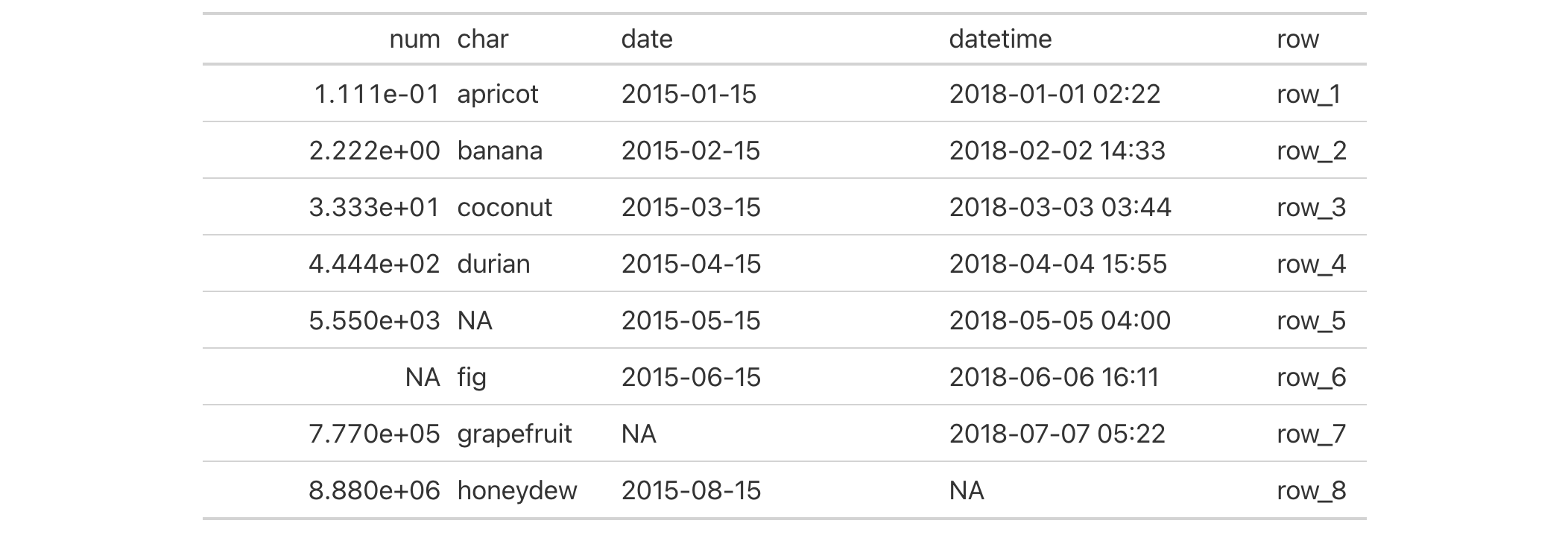# cols_width: Set the widths of columns In gt: Easily Create Presentation-Ready Display Tables

 cols_width R Documentation

## Set the widths of columns

### Description

Manual specifications of column widths can be performed using the `cols_width()` function. We choose which columns get specific widths. This can be in units of pixels (easily set by use of the `px()` helper function), or, as percentages (where the `pct()` helper function is useful). Width assignments are supplied in `...` through two-sided formulas, where the left-hand side defines the target columns and the right-hand side is a single dimension.

### Usage

```cols_width(.data, ..., .list = list2(...))
```

### Arguments

 `.data` A table object that is created using the `gt()` function. `...` Expressions for the assignment of column widths for the table columns in `.data`. Two-sided formulas (e.g, ` ~ `) can be used, where the left-hand side corresponds to selections of columns and the right-hand side evaluates to single-length character values in the form `{##}px` (i.e., pixel dimensions); the `px()` helper function is best used for this purpose. Column names should be enclosed in `c()`. The column-based select helpers `starts_with()`, `ends_with()`, `contains()`, `matches()`, `one_of()`, and `everything()` can be used in the LHS. Subsequent expressions that operate on the columns assigned previously will result in overwriting column width values (both in the same `cols_width()` call and across separate calls). All other columns can be assigned a default width value by using `everything()` on the left-hand side. `.list` Allows for the use of a list as an input alternative to `...`.

### Details

Column widths can be set as absolute or relative values (with px and percentage values). Those columns not specified are treated as having variable width. The sizing behavior for column widths depends on the combination of value types, and, whether a table width has been set (which could, itself, be expressed as an absolute or relative value). Widths for the table and its container can be individually modified with the `table.width` and `container.width` arguments within `tab_options()`).

### Value

An object of class `gt_tbl`.

### Examples

Use `exibble` to create a gt table. We can specify the widths of columns with `cols_width()`. This is done with named arguments in `...`, specifying the exact widths for table columns (using `everything()` at the end will capture all remaining columns).

```exibble %>%
dplyr::select(
num, char, date,
datetime, row
) %>%
gt() %>%
cols_width(
num ~ px(150),
ends_with("r") ~ px(100),
starts_with("date") ~ px(200),
everything() ~ px(60)
)
```### Function ID

4-3

Other column modification functions: `cols_align_decimal()`, `cols_align()`, `cols_hide()`, `cols_label()`, `cols_merge_n_pct()`, `cols_merge_range()`, `cols_merge_uncert()`, `cols_merge()`, `cols_move_to_end()`, `cols_move_to_start()`, `cols_move()`, `cols_unhide()`